Optimization
General Enhancements/Fixes to Optimization
• Checks were added for errors returned from CPLEX function calls. Diagnostics will now be able to notify the user of errors and warnings that occur in CPLEX functions.
• The default value of minimax optimally was changed from 1x10-3 to 1x10-6.
• A new function was added to check approximation quality for both 2pt-line and piece wise calculations.
• The behavior of the Pumped Storage Reservoir is now consistent with the other reservoir objects when it is included in the No Opt Subbasin. This means that the Opt In column for Outflow and Pumped Flow is used to estimate flows which will affect optimized objects. These values are NOT used in simulation. The assumption is that the operation will be set using some combination of simulation columns. Alternatively, Outflow and Pumped Flow could just be entered as inputs in the simulation column.
• A function was added to add up individual spill requirements and put them in the Spill Opt In column. These values are used as lower bounds on Spill.
• An additional row was added to the Total Values slot. The row contains the simulation value of the optimization objective, which is the avoided cost plus the cumulative value of storage. This value is also reported to the diagnostics window.
• The marginal cost calculations were changed. If a unit or block exactly covers the load or generation then the marginal cost reported is the next unit or block that would be used.
Smooth Energy Calculations
Purpose
The smooth energy calculations were developed to deal with the way optimization handles energy production among reservoirs. The current optimization finds extreme points of an optimal region, where the production of energy is moved from reservoir to reservoir and from timestep to timestep in a disjoint manner. An example of this problem is the following simple matrix:

Timestep 1
Timestep 2
TOTAL
Reservoir 1
10
0
10
Reservoir 2
0
20
20
TOTAL
10
20

In most practical cases, the energy demands at every timestep are almost constant, and the energy supply from every reservoir is also almost constant over the run. There are many ways to allocate the energies, but this extreme case is not good. Instead, it is desirable that a smoother energy allocation is found, where each reservoir at each timestep contributes a proportionate amount to satisfying the demand.
Results
Ideally, the energy at each reservoir for each timestep will have a policy constraint at a priority p which is directly after the max avoided cost objective. The following constraint can be written to result in “smoothed” energy values:
(34.1)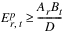The constraint defined by equation 1 is written for some t, where Ep is the energy (actual variable) at the subscripted reservoir and timestep,
(34.2)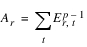where Ep-1 is the resultant energies from the last priority level, and the r subscript is constant,
(34.3)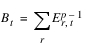where Ep-1 is the resultant energies from the last priority level, and the t subscript is constant and
(34.4)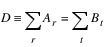Equation 1 is a simplified constraint and does not include any flexibility terms. However for the simple example in Table 1, Equation 1 can be applied to get the following results:

Timestep 1
Timestep 2
TOTAL
Reservoir 1
10/3
20/3
10
Reservoir 2
20/3
40/3
20
TOTAL
10
20

example for row 1, column 1:
(34.5)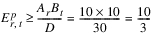The optimization will attempt to get as close to the results in Table 2 as possible. In the case where there may be flexibility terms, the total values are allowed to vary slightly.
Policy Constraints
Because the interface to the user is the constraint editor, the trigger for the creation of the constraints listed above must be simple. This is done through the use of a special subbasin name, Smooth Energy Reservoirs, to which users will add the reservoirs used in the smooth energy calculations. Then, the user will write a single policy constraint: for [t in time by <slot>, ThermalObject.Energy Result[t] = ThermalObject.Smooth Energy[t]]
This constraint will be replaced with a constraint for each of the reservoirs for each timestep. The slots referenced in the user constraint above will be slots that are flagged as linearized variables. The Energy Result slot will be ignored while linearization of the Smooth Energy will trigger the writing of all the physical and policy constraints.
Revised: 08/02/2021